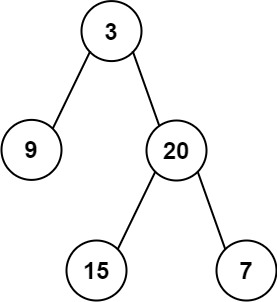# GeetCode Hub

Given the `root` of a binary tree, return the sum of all left leaves.

Example 1:```Input: root = [3,9,20,null,null,15,7]
Output: 24
Explanation: There are two left leaves in the binary tree, with values 9 and 15 respectively.
```

Example 2:

```Input: root = 
Output: 0
```

Constraints:

• The number of nodes in the tree is in the range `[1, 1000]`.
• `-1000 <= Node.val <= 1000`

/** * Definition for a binary tree node. * public class TreeNode { * int val; * TreeNode left; * TreeNode right; * TreeNode() {} * TreeNode(int val) { this.val = val; } * TreeNode(int val, TreeNode left, TreeNode right) { * this.val = val; * this.left = left; * this.right = right; * } * } */ class Solution { public int sumOfLeftLeaves(TreeNode root) { } }